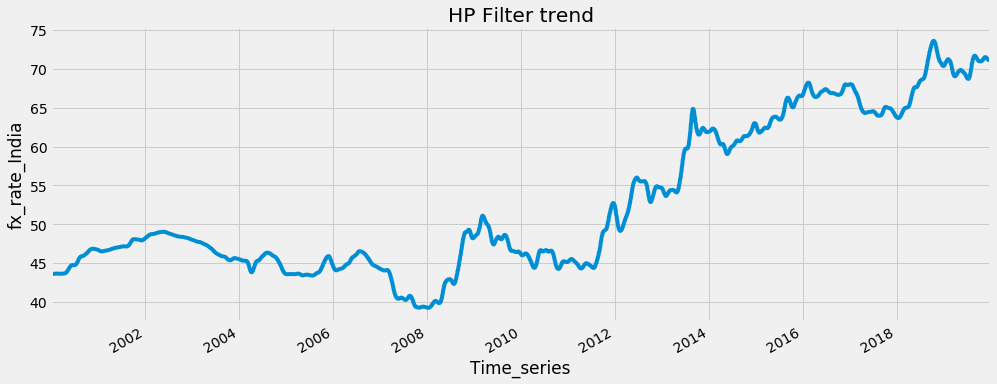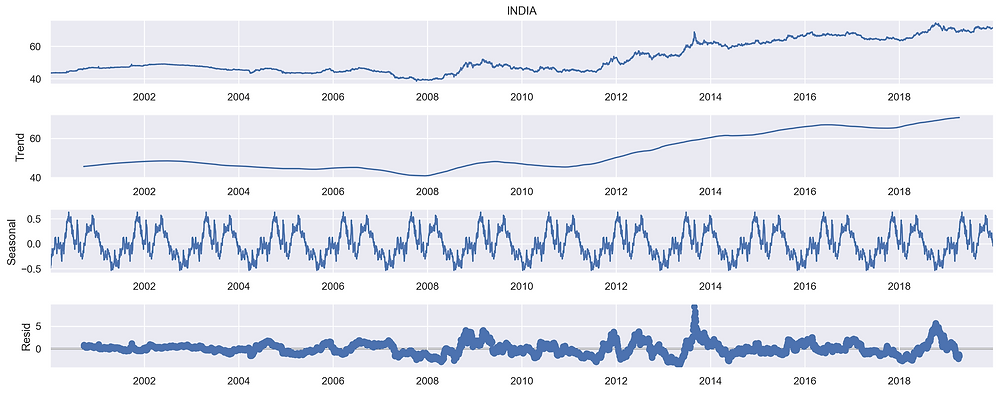Search
•SoniaSamipillai

# Time Series Analysis - Part 1 - Understanding Time Series Data“The only reason for time is so that everything doesn't happen at once.” “Time and space are modes by which we think and not conditions in which we live.” “The distinction between the past, present and future is only a stubbornly persistent illusion.”

As a data person, you get to deal with different types of data. Data in itself can be classified in many different angles. On a broader perspective, we classify data into qualitative and quantitative. Quantitative data can be counted, measured and expressed using numbers. Qualitative data is descriptive and conceptual. In yet another classification, data can be classified as Nominal, Ordinal, Ratio and Interval based on the scale that is used for collecting data. Data can also be classified broadly into Time Series data, Cross-Sectional data and Panel data.

Cross-Section Data

Cross-sectional data can comprise of observations taken at different points in time, however, in such cases time itself does not play any significant role in the analysis. Analysis of cross-sectional data usually consists of comparing the differences among selected subjects. Examples of cross section data are GDP published by IMF for June 2018 and GRE scores of students in 2018.

Panel or Longitudinal Data

Observations on multiple phenomena over multiple time periods is called Panel data. Panel data combines both cross-sectional and time series data ideas and looks at how the subjects (firms, individuals, etc.) change over a time series.

Time- Series data

When data is collected and stored over a period of time, we call it Time Series. Every data point is recorded against a specific time and when this data is mined for patterns and insights, it is called Time-Series Analysis. Statisticians observe the trend of the data in the past for an accumulated period of time and try to forecast the future. Some of the examples of time series data are IOT(data from Internet Of Things), sensors data, stock market, weather data and so on. In this blogpost, we will discuss about Time series data and its characteristics.

Univariate or Multivariate

A time series contains data points that increase, decrease, or otherwise change in chronological order over a period. A time series that incorporates the records of a single feature or variable is called a univariate time series. If the records incorporate more than one feature or variable, the series is called a multivariate time series.

Continuous or Discrete

A discrete signal or discrete-time signal is a time series consisting of a sequence of quantities as occurring at distinct, separate "points in time". A continuous signal or a continuous-time signal is a varying quantity and is a continuum in time domain .Any analog signal is continuous by nature. Natural processes occurs in continuous fashion over an interval of time and space. We look at a particular variable, not at a point in time, rather at a set of times. Hence look at a signal as a function of time, f(t) and examples of f(t) might be price of a stock at time t or speed of a vehicle at time t .

So a discrete time signal is discrete only in time domain and not in amplitude. It can become discrete in amplitude only after quantization. A quantized discrete time signal is called digital signal. Discrete-time signals, used in digital signal processing, can be obtained by sampling and quantization of continuous signals. Quantization is nothing but the process of constraining an input from a continuous or otherwise large set of values (such as the real numbers) to a discrete set (such as the integers).

In a continuous time series, data observation is carried out continuously throughout the period, as with earthquake seismograph magnitude data. In a discrete time series , data observation is carried out at a specific time or equally spaced, as with temperature increases or decreases, exchange rates of currencies, air pressure data and so on.

Now that we discussed about the signal as a function of time, it is to be noted that if there is one dependent variable in a signal, the signal is considered one dimensional where time is the independent variable and the value f(t)(aka amplitude) is the dependent variable. In case of image as signal, it is two dimensional f(x, y), the two independent variables are width and height, and the dependent variable f is also called intensity.

Characteristics of Time Series data

1. Trend

2. Seasonality

3. Cyclical Variations

4. Errors, Residuals or Unexpected variations

Trend

A trend is a pattern that is observed over a period of time . Its the mean rate of change with respect to time. Trend represents a source of variability. Trend does not have to increase or decrease in the same direction. That is both the direction and slope(rate of change) may remain constant or might change. Because a trend in the data represents a significant source of variability, it must be accounted for when performing any time series analysis. That is, it must be either (a) modeled explicitly or (b) removed through mathematical transformations . The former approach is taken when the trend is theoretically interesting—either on its own or in relation to other variables. Conversely, removing the trend is performed when this component is not pertinent to the goals of the analysis. The decision of whether to model or remove systematic components like a trend represents an important aspect of time series analysis.Detect Trend

Trend can be detected using HP(Hodrick prescott) filter. This technique dissolves the time series into trend and seperates the trend from the cyclical components. Using HP filter on the above data, we obtain the trend that is displayed below.Seasonality

Seasonality is a pattern that occurs regularly over a period of time. This mainly occurs with sales data , stock market, weather and a characteristic of the economics. It doesnt occur often in scientific data.

Detect Seasonality

Multiple box plots can be plotted to check for seasonality. a box plot depicts the spread of data over a range. It shows the minimum, first Quartile, Second Quartile, Third Quartile and maximum. Look at this multi-month wise box plot for the foreign exchange rate for India. The plots are observed for shifts in location, variation and outliers. Most of the months seem to have the mean around 45-50. April has a higher rate compared to the rest of the months. When reading the box plot, look for

1. Is a factor significant?

2. Does the location differ between subgroups?

3. Does the variation differ between subgroups?

4. Are there any outliers?Cyclical Variations, Errors, Residuals or Unexpected variations

Cyclical variations are recurring fluctuations less frequent than seasonality. It can happen due to economic variations like prosperity, depression or accessibility

Detect Cyclical Variations

Cyclical variations can be detected by HP filter. The cyclical variation for the India exchange rate is shown below.When trend and seasonality are removed from time-series data, the patterns left behind that cannot be explained are called errors, unexpected variations, or residuals. Various methods are available to check for irregular variations such as probability theory, moving averages, and autoregressive time-series methods. If we can find any cyclic variation in data, it is considered to be part of the residuals. These variations that occur due to unexpected circumstances are called unexpected variations or unpredictable errors.

Decompose Time Series into its components

Stats models provides seasonal_decompose module that decomposes the time series into the four main components trend, seasonality, cyclical variations and errors, using additive model and multiplicative model. Additive model is given by

`y[t] = T[t]+s[t]+c[t]+e[t]`

The additive model works with linear trends of time-series data such as changes constantly over time. The variable we have been looking so far has the linear trend.

The multiplicative model works with a nonlinear type of data such as quadric or exponential. Multiplicative model is given by

`y[t] = T[t]*s[t]*c[t]*e[t]`

The primary purpose of time series decomposition is to provide the analyst with a better understanding of the underlying behavior and patterns of the time series which can be valuable in determining the goals of the analysis.Seasonal decompose using multiplicative modelThe additive decomposition model is most appropriate when the magnitude of the trend-cycle and seasonal components remain constant over the course of the series. However, when the magnitude of these components varies but still appears proportional over time (i.e., it changes by a multiplicative factor), the series may be better represented by the multiplicative decomposition model, where each observation is the product of the trend-cycle, seasonal, and random components.

The notebook used for this analysis can be found here.

Hope this post was helpful!!. If you’re interested to read more, please subscribe and be notified when the next article is published.